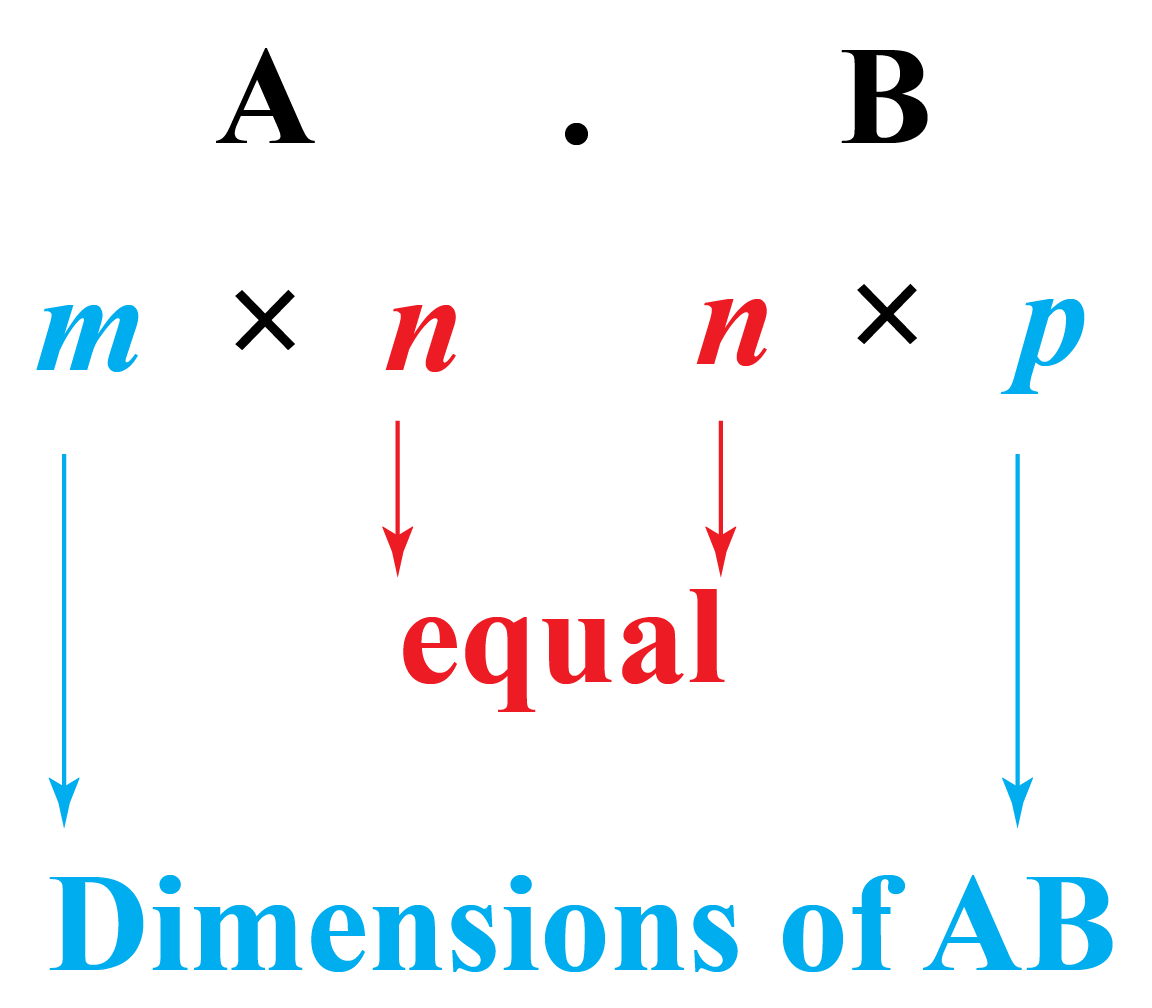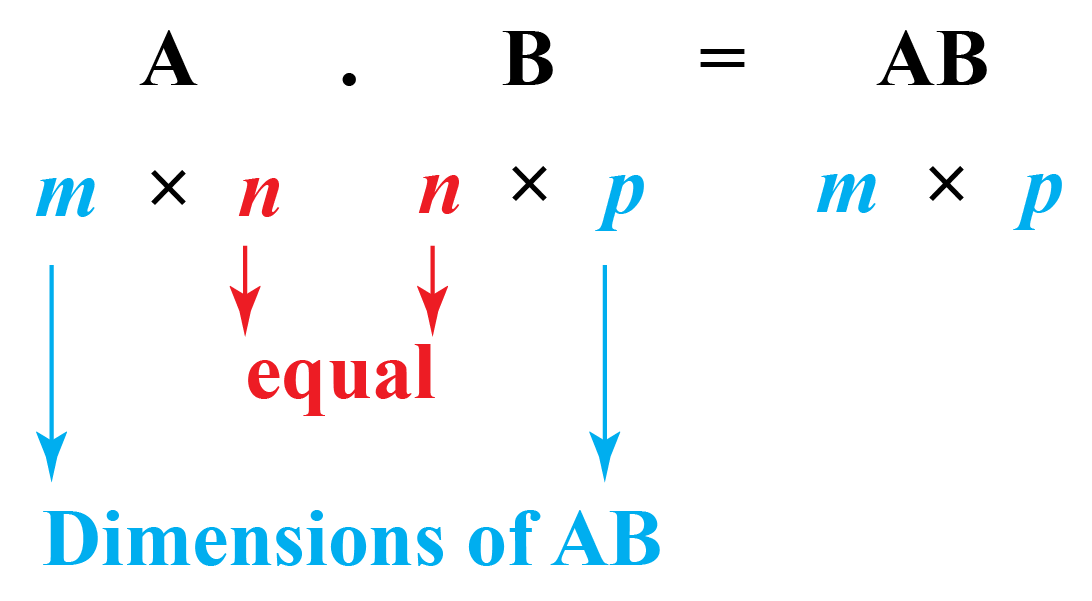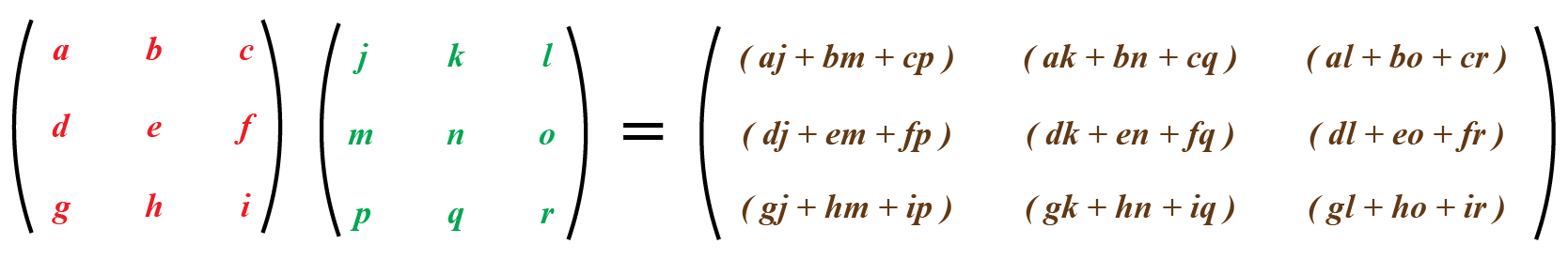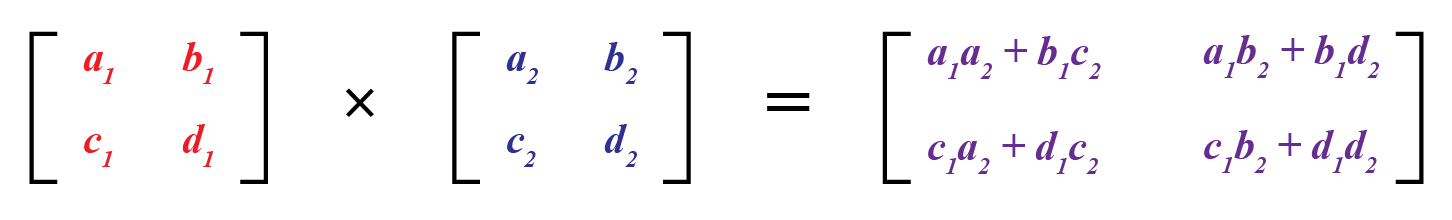# Multiplication of Matrices

Multiplication of Matrices

Are matrix multiplication and normal multiplication really similar?

Except for the name, they both differ in the process as well as in properties.

Let us look at the following scenario.

Two school cricket teams submit equipment lists for the season.

The cost of each bat, ball, and uniform (blazer) is $20,$5, and \$28 respectively.

The girls's team requires 10 bats, 40 balls, and 12 blazers, and the boys's team requires 12 bats, 48 balls, and 11 uniforms.

Find the total cost of the equipment.

Generally, to find the total cost, we opt for the preferred method of forming equations and solving them.

In this short lesson, let us try to solve similar problems with the help of matrices and explore a new way of finding the cost using matrix multiplication.

Here is an interactive calculator for the multiplication of matrices. Try it for a hands-on experience with the matrix multiplication calculator!

Input the order of the matrices A and B and press GENERATE MATRICES.

Then enter the values accordingly and press ENTER to obtain the product of those two matrices.

## Lesson Plan

 1 What Is the Meaning of Matrix Multiplication? 2 Thinking Out of the Box! 3 Solved Examples on Multiplication of Matrices 4 Important Notes on Multiplication of Matrices 5 Interactive Questions on Multiplication of Matrices

## What Is the Meaning of Matrix Multiplication?

Matrix multiplication is a binary operation whose output is also a matrix when two matrices are multiplied.

### Is Matrix Multiplication Always Defined?

Matrix multiplication is possible only when the matrices are compatible.

### Compatibility of Matrices:

Two matrices A and B are said to be compatible if the number of columns in A is equal to the number of rows in B.

That means if A is a matrix of order m$$\times$$n and B is a matrix of order n$$\times$$p, then we can say that matrices A and B are compatible.### The Rule for Matrix Multiplication:

If A is a matrix of order m$$\times$$n and B is a matrix of order n$$\times$$p, then the order of the product matrix is m$$\times$$p.For example, a) Multiplying a 4 × 3 matrix by a 3 × 4 matrix is valid and it gives a matrix of order 4 × 4

b)  7 × 1 matrix and 1 × 2 matrices are compatible; the product gives a 7 × 2 matrix.

c) Multiplication of a 4 × 3 matrix and 2 × 3 matrix is NOT possible.

## What Is the Matrix Multiplication Formula?

We can understand the general process of matrix multiplication by the technique, "First rows are multiplied by columns (element by element) and then the rows are filled up."

Consider two matrices of order 3*3

$\begin{pmatrix} a & b & c\\ d & e & f\\ g & h & i \end{pmatrix} % \begin{pmatrix} j & k & l\\ m & n & o\\ p & q & r \end{pmatrix}$

Here, the matrices have the same dimensions, so the resultant matrix will also have the same dimension 3*3General steps in matrix multiplication:

1. Make sure that the number of columns in the 1st matrix equals the number of rows in the 2nd matrix (compatibility of matrices).

2. Multiply the elements of each row of the first matrix by the elements of each column in the second matrix.

3. Add the products.

4. Place the added products in the respective columns.

## What Is the 2*2 Matrix Multiplication?

The process is the same for the matrix of any order.

We multiply the elements of each row of the first matrix by the elements of each column in the second matrix (element by element).

Finally, we add the products.## What Is the 3*3 Matrix Multiplication?

The process is exactly the same for the matrix of any order.Think Tank
1. By using the matrices shown below, check whether matrix multiplication is commutative or not. $\begin{pmatrix} 1 & 0 \\ 2 & 4 \\ \end{pmatrix} \text{and}\begin{pmatrix} 6 & 8 \\ 4 & 3 \\ \end{pmatrix}$
2. Is matrix multiplication associative?

## Solved Examples

 Example 1

Find the product of the matrices:

$\begin{pmatrix} 1 & 0 \\ 2 & 4 \\ \end{pmatrix} \text{and}\begin{pmatrix} 6 & 8 \\ 4 & 3 \\ \end{pmatrix}$

Solution

The given matrices are of order 2*2

They are compatible and the product matrix will also be 2*2

\begin{equation}
\text {Product of matrices  }\left(\begin{array}{ll}1 & 0 \\ 2 & 4\end{array}\right) \text { and }\left(\begin{array}{ll}6 & 8 \\ 4 & 3\end{array}\right) \text { is: }
\end{equation}

\begin{eqnarray}
&&\begin{pmatrix}
1 & 0 \\
2 & 4 \\
\end{pmatrix}.\begin{pmatrix}
6 & 8 \\
4 & 3 \\
\end{pmatrix}\\\\
&=& \begin{pmatrix}
(1\times6)+(0\times4) & (1\times8)+(0\times3) \\
(2\times6)+(4\times4) & (2\times8)+(4\times3) \\
\end{pmatrix} \\\\ &=& \begin{pmatrix}
6+0 && 8+0 \\
12+16&& 16+12 \\
\end{pmatrix}
\\\\
&=& \begin{pmatrix}
6 & 8 \\
28& 28 \\
\end{pmatrix}
\end{eqnarray}

 $$\therefore$$ Product matrix is $\begin{pmatrix} 6 & 8 \\ 28 & 28 \\ \end{pmatrix}$
 Example 2

There are three ways to travel from City A to City B: car, train, and plane.

The travel fee is different for adults and children and the prices are different for journeys in the morning and afternoon.

Below are two tables summarizing the total cost of the trip during the mornings and afternoons.

ADULT Car Train Plane
Morning fare 200 160 235
Afternoon fare 250 150 270

CHILD Car Train Plane
Morning fare 140 110 185
Afternoon fare 200 120 160

Represent the information using the cost matrix for adult and child.

Solution

We can represent the above information with the help of two matrices.

Rows: by car, by train, by plane

Columns: adult, child

The fare in the morning is shown as Matrix M: $\begin{pmatrix} 200 & 140 \\ 160 & 110 \\235 & 185 \end{pmatrix}$

The fare in the evening is shown as Matrix N:$\begin{pmatrix} 250 & 200 \\ 150 & 120 \\270 & 160 \end{pmatrix}$

 $$\therefore$$ M and N represent the respective cost matrices.
 Example 3

Matrix A is of 4$$\times$$1 order.

Matrix B is of 1$$\times$$3 order.

Are both the products  AB and BA defined?

Solution

Matrix A is of order 4$$\times$$1

Matrix B is of order 1$$\times$$3

The product AB is defined as the number of columns of A is the same as the number of rows of B.

The product matrix hence obtained is of order 4$$\times$$3

The product BA is not defined as the number of columns of B is not equal to the number of rows of A.

So, we can say that matrix multiplication is not commutative, AB is not necessarily equal to BA and sometimes one of the products may not be defined also.

 $$\therefore$$ Product AB is defined but not BA.
 Example 4

Find the product of $\begin{pmatrix} 1 & 2 \\ 3 & 4 \\ 5 & 1 \\ \end{pmatrix} \text{and}\begin{pmatrix} 2 \\ 4 \\ \end{pmatrix}$

Solution

The given matrices are of order 3*2 and 2*1

They are compatible and the product matrix will be of order 3*1

\begin{eqnarray}
&&\begin{pmatrix}
1 & 2 \\
3 & 4 \\ 5 & 1 \\
\end{pmatrix}.\begin{pmatrix}
2 \\
4 \\
\end{pmatrix}\\\\
&=& \begin{pmatrix}
(1\times2)+(2\times4) \\
(3\times2)+(4\times4) \\ (5\times2)+(1\times4) \\
\end{pmatrix} \\\\ &=& \begin{pmatrix}
2+8 \\
6+16 \\ 10+4 \\
\end{pmatrix}
\\\\
&=& \begin{pmatrix}
10 \\
22 \\14\\
\end{pmatrix}
\end{eqnarray}

 $$\therefore$$Product matrix is $\begin{pmatrix} 10 \\ 22\\14 \\ \end{pmatrix}$

## Let's Summarize

The mini-lesson targeted the fascinating concept of the multiplication of matrices. The math journey around the multiplication of matrices started with what a student already knew and went on to creatively crafting a fresh concept in the young minds. Done in a way that not only it is relatable and easy to grasp, but also will stay with them forever.

## About Cuemath

At Cuemath, our team of math experts is dedicated to making learning fun for our favorite readers, the students!

Through an interactive and engaging learning-teaching-learning approach, the teachers explore all angles of a topic.

Be it worksheets, online classes, doubt sessions, or any other form of relation, it’s the logical thinking and smart learning approach that we, at Cuemath, believe in.

More Important Topics
Numbers
Algebra
Geometry
Measurement
Money
Data
Trigonometry
Calculus